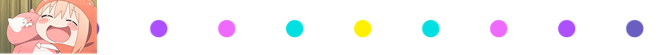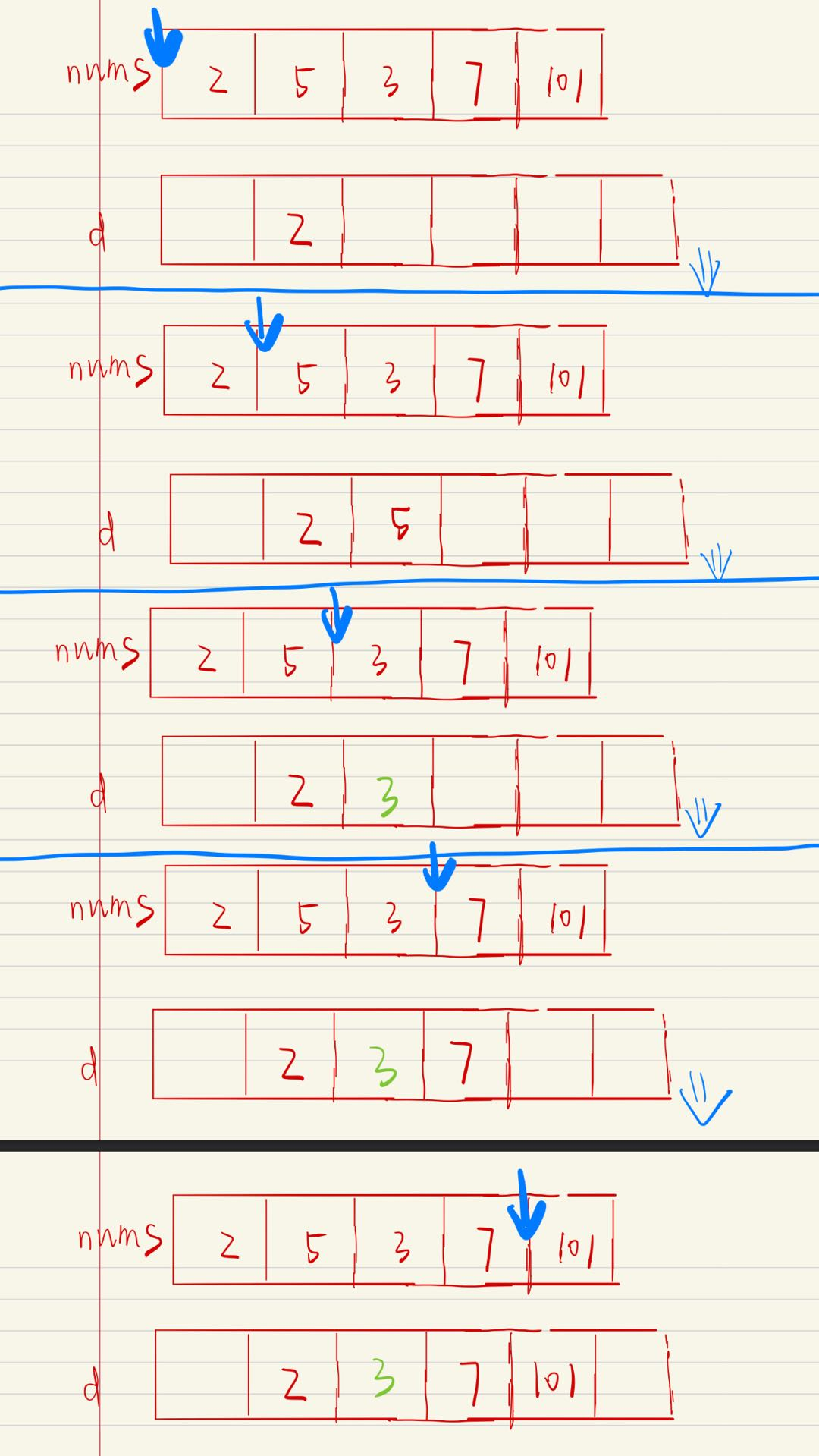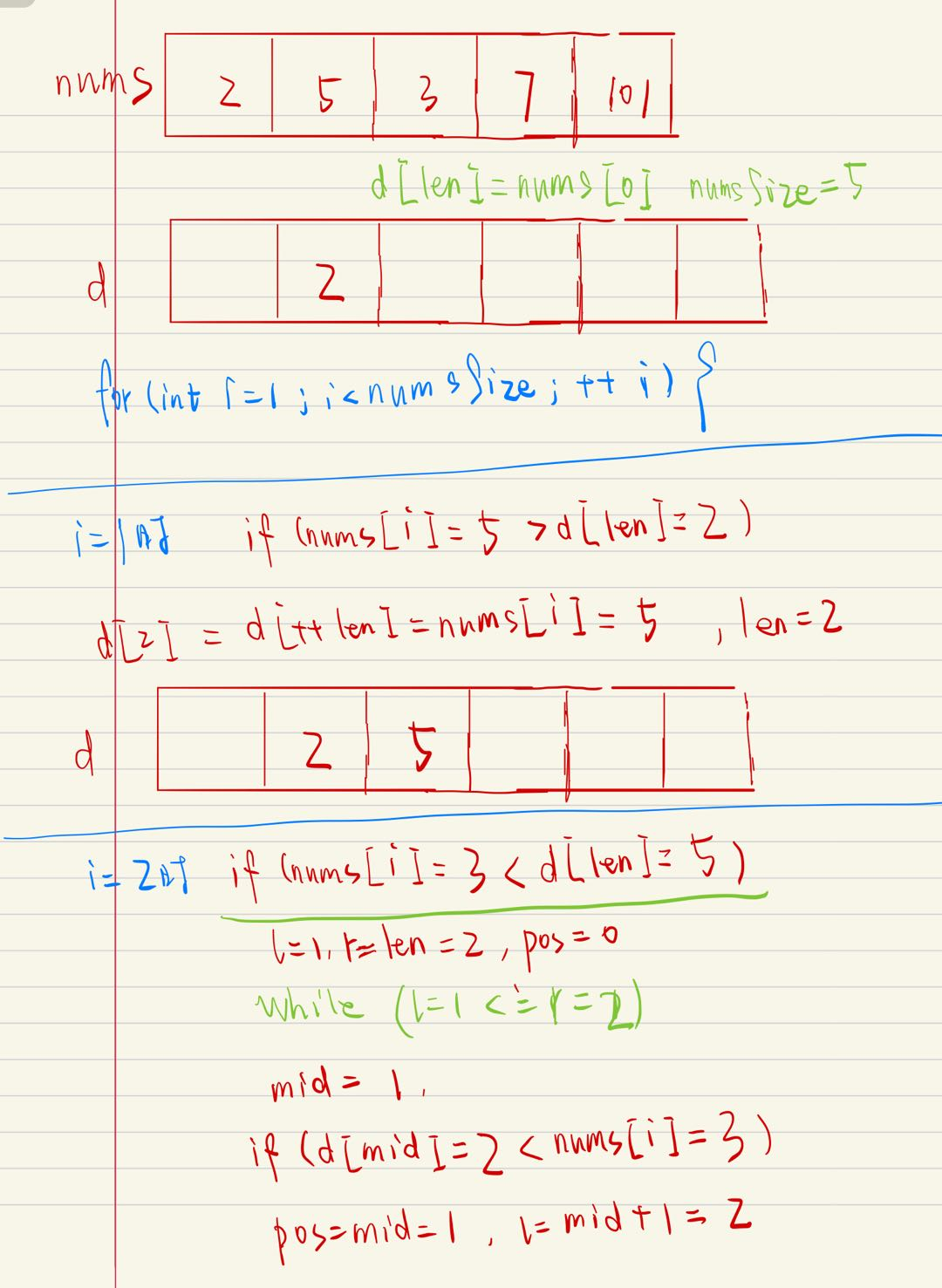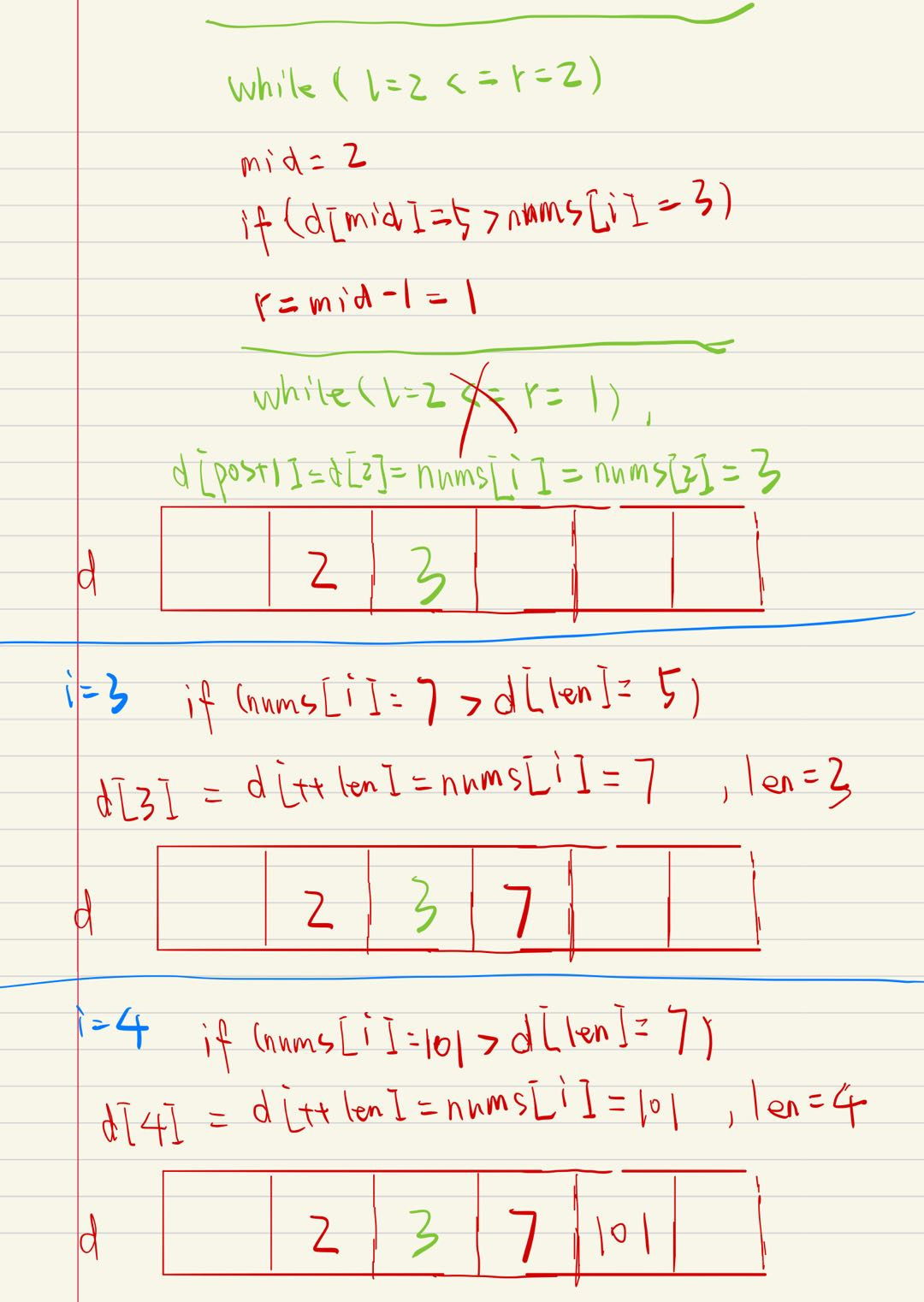# 【手绘漫画】图解LeetCode之最长上升子序列（LeetCode300题），贪心算法 + 二分查找# 图解LeetCode刷题计划

## 1、写在前面## 2、题目## 3、正文• 时间复杂度： O ( n l o g n ) O(nlogn) 。数组 nums \textit{nums} 长度 n，二分搜索是 O ( log ⁡ n ) O(\log n)
• 空间复杂度： O ( n ) O(n) ，长度为 n 的数组 d## 4、代码

int lengthOfLIS(int* nums, int numsSize){
int len = 1;
if (numsSize == 0) return 0;
int d[numsSize+1];
d[len] = nums;
for (int i = 1; i < numsSize; ++i) {
if (nums[i] > d[len]){
d[++len] = nums[i];
}
else{
int l = 1, r = len, pos = 0;
while (l <= r) {
int mid = l + (r - l)/2;
if (d[mid] < nums[i]) {
pos = mid;
l = mid + 1;
}
else {
r = mid - 1;
}
}
d[pos + 1] = nums[i];
}
}
return len;
}## 5、讨论

1、严格意义上，这个题和二分查找没啥特别大的关系，23333。2、为什么数组 d 要是 d[numsSize+1]?

3、数组 d 是单调递增的嘛？05-02173
06-06135507-20112
03-1479
04-15601
06-03114
11-30233
09-15441
08-0931